# ReInceptionE: Relation-Aware Inception Network with Joint Local-Global Structural Information for KGE

## Basic Idea

1. ConvE中没有融入结构化信息.
2. ConvE的性能仍然被交互次数所限制.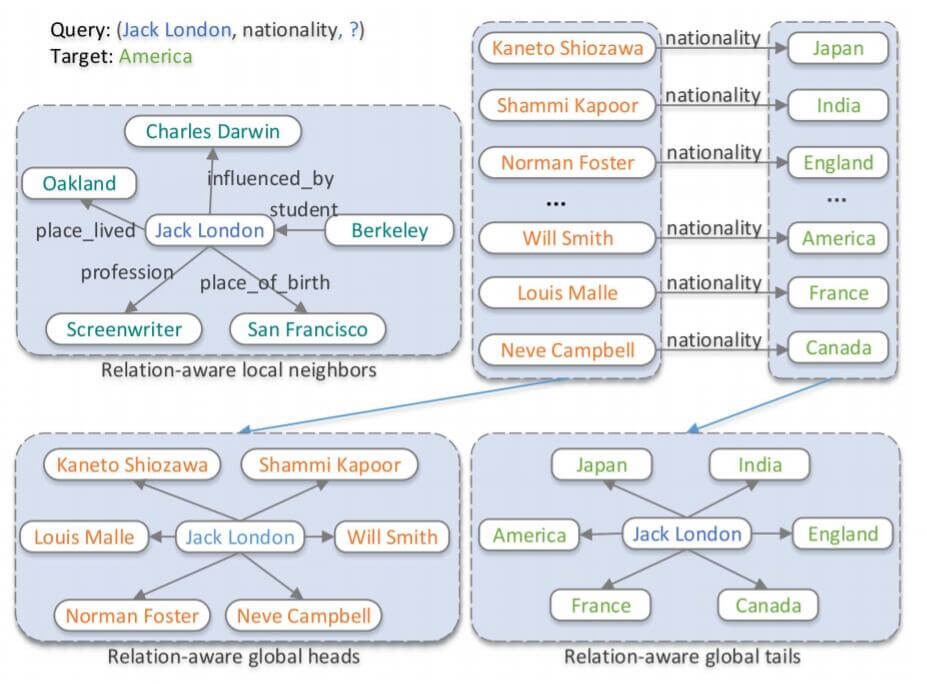## ReInceptionE

ReInceptionE(Relation - aware Inception network with joint local-global structural information for knowledge graph Embedding) 将Inception和KBAT的优势结合到了一起, 作者先用Inception在实体和关系之间交互, 生成头实体和关系之间的查询向量, 然后从局部全局两个角度集成信息, 用Attention把信息融合到一起.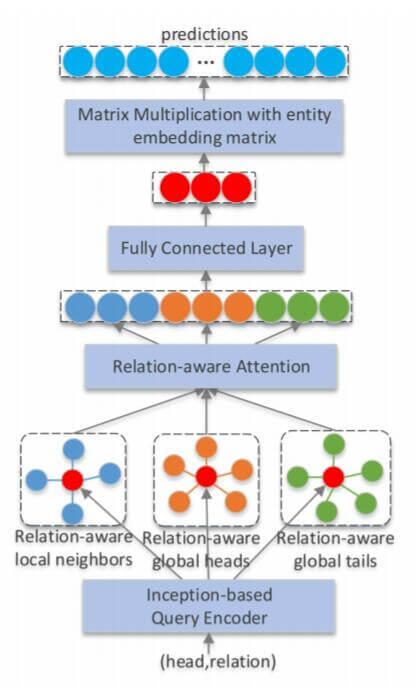### Inception - based Query Encoder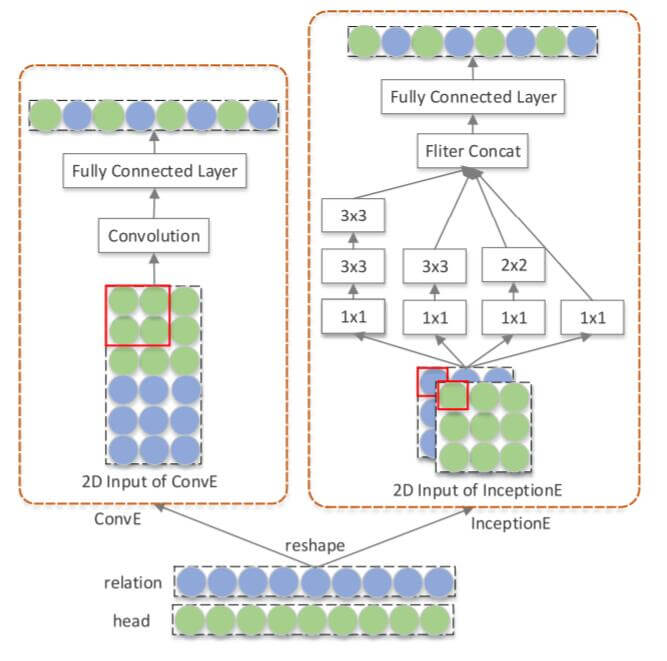CoPER也是针对这个点改进的, 只不过使用的是参数生成的方法, 避免了Concatenate这种加性操作.

$$\mathbf{v}_{1 \times 1}=\operatorname{Relu}\left(\left[\mathbf{v}_{h} \lVert\mathbf{v}_{r}\right] \ast \omega_{1 \times 1}\right)$$

$$\mathbf{v}_{2(3 \times 3)}=\operatorname{Relu}\left(\operatorname{Relu}\left(\mathbf{v}_{1 \times 1}^{2(3 \times 3)} \ast \omega_{3 \times 3}^{1}\right) \ast \omega_{3 \times 3}^{2}\right)$$

$\mathbf{v}_{1 \times 1}^{2(3 \times 3)}$ 为输入的交互特征, 即$1\times1$ 卷积后的结果, $\omega_{3\times3}^1, \omega_{3\times3}^2$ 分别为两个$3\times3$ 的卷积核参数.

\begin{aligned} \mathbf{v}_{q} &=\text { Inception }\left(\mathbf{v}_{h}, \mathbf{v}_{r}\right) \\ &=\operatorname{Relu}\left(\operatorname{vec}\left(\left[\mathbf{v}_{1 \times 1}\lVert \mathbf{v}_{2 \times 2} \lVert \mathbf{v}_{3 \times 3} \lVert \mathbf{v}_{2(3 \times 3)}\right]\right) \mathbf{W}\right) \end{aligned}

$\mathbf{W}$ 为一层FC的参数.

### Relation - Aware Local Attention

$$\mathbf{v}_{n_{i}}=\operatorname{Inception}\left(\mathbf{v}_{e_{i}}, \mathbf{v}_{r_{i}}\right)$$

$$s_{i}=\operatorname{LeakyRelu}\left(\mathbf{W}_{1}\left[\mathbf{W}_{2} \mathbf{v}_{q} \lVert \mathbf{W}_{3} \mathbf{v}_{n_{i}}\right]\right)$$

$$\alpha_{i}=\frac{\exp \left(s_{i}\right)}{\sum_{n_{j} \in \mathcal{N}_{a}} \exp \left(s_{j}\right)}$$

$$\mathbf{v}_{n}=\operatorname{Relu}\left(\sum_{n_{i} \in \mathcal{N}_{q}} \alpha_{i} \mathbf{W}_{3} \mathbf{v}_{n_{i}}\right)+\mathbf{W}_{2} \mathbf{v}_{q}$$

$$\mathbf{v}_{n}=Re A t t\left(\mathbf{V}_{n}, \mathbf{v}_{q}\right)$$

$\mathbf{V}_n = \left\{ \mathbf{v}_n \mid n_i \in \mathcal{N}_q \right\}$ 为局部邻居向量的集合.

### Relation - Aware Global Attention$$\mathbf{v}_{r h}=Re A t t\left(\mathbf{V}_{r h}, \mathbf{v}_{q}\right)$$

$\mathbf{V}_{rh}=\left\{\mathbf{v}_{h_{ri}}\mid h_{ri} \in \mathcal{H}_r\right\}$ 是对应关系中的所有头实体集合.
$$\mathbf{v}_{r t}=ReAtt\left(\mathbf{V}_{r t}, \mathbf{v}_{q}\right)$$

$\mathbf{V}_{rt}=\left\{\mathbf{v}_{t_{ri}}\mid t_{ri} \in \mathcal{T}_r\right\}$ 是对应关系中的所有尾实体集合.

### Joint Relation - Aware Attention

$$\mathbf{v}_{q}^{\prime}=\mathbf{W}_{4}\left[\mathbf{v}_{n}\lVert\mathbf{v}_{r h} \lVert \mathbf{v}_{r t}\right]+\mathbf{b}$$

$\mathbf{W}_4, \mathbf{b}$ 为权重矩阵和偏置项.

$$f(h, r, t)=\mathbf{v}_{q}^{\prime T} \mathbf{v}_{t}$$

$$p(t\mid h, r)=\frac{\exp (\lambda f(h, r, t))}{\sum_{\left(h, r, t^{\prime}\right) \in \mathcal{G}^{\prime} \cup\{(h, r, t)\}}\exp \left(\lambda f\left(h, r, t^{\prime}\right)\right)}$$

$\lambda$ 为平滑参数, $\mathcal{G}^\prime$ 为负采样获得的三元组集合.

$$\mathcal{L}=-\frac{1}{|\mathcal{E}|} \sum_{i=0}^{|\mathcal{E}|} \log p\left(t_{i} \mid h_{i}, r_{i}\right)$$

$\mathcal{E}$ 为$\mathcal{G}$ 中的有效实体总数.

## Experiments

### Main Results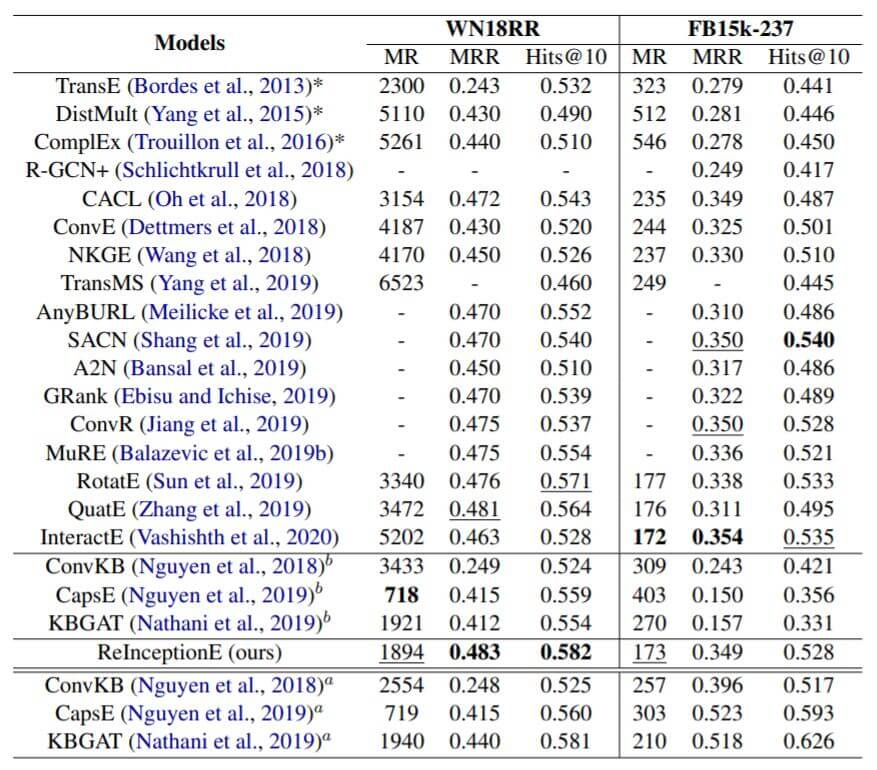Baseline有很多是基于图或基于CNN的方法. 右上角角标b代表从另一篇论文重新运行代码得出的结果, a代表作者从原论文中得到的结果.

ConvKB, CapsE, KBGAT的评估协议是不公平的, 我建议不要关注这三个模型的结果.

ReInceptionE其实也并没有超出其他Baseline很多的性能, 感觉效果其实差不多.

### Impact of Different Modules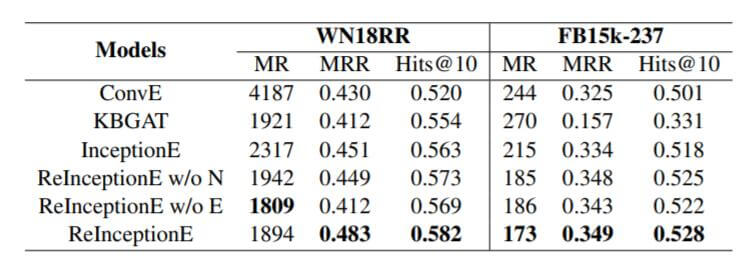ReInception w/o N是模型不使用局部信息, ReInception w/o E是不使用全局信息. 单独使用InceptionE的效果就比ConvE好很多, 在加入局部信息后, 效果比KBAT也有改善. 与只使用局部信息相比, 只使用全局信息更不利于模型区分三元组. 二者结合的情况下, 效果最佳.

### Evaluation on Different Relation Types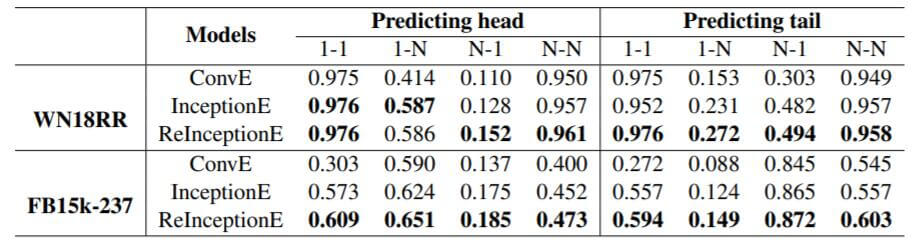### Case Study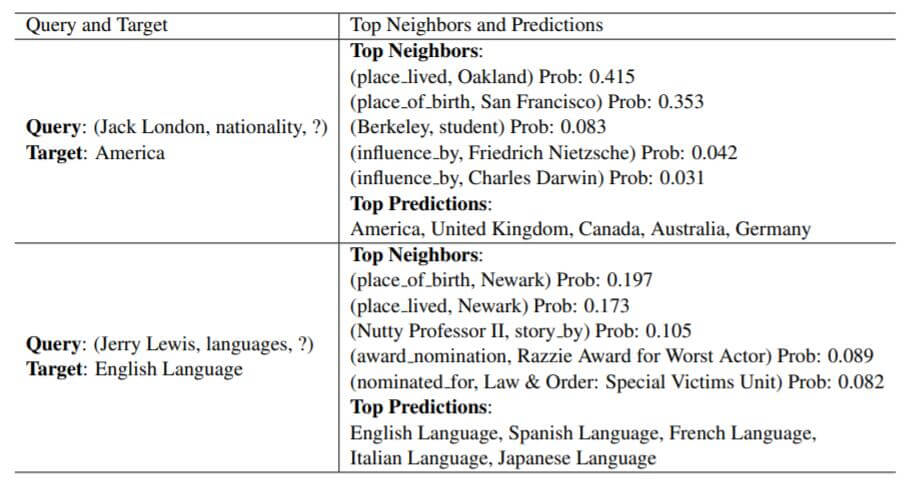## Summary

ReInceptionE使用Inception作为增加实体与关系交互的基本组件, 并在KBAT的基础上集成局部全局信息, 用Relation Aware Attention的方式将二者融合到一起,

上一篇HAKE: Learning Hierarchy-Aware Knowledge Graph Embeddings for Link Prediction

2021-04-11KBAT: Learning Attention-based Embeddings for Relation Prediction in KGs

2021-04-04
目录